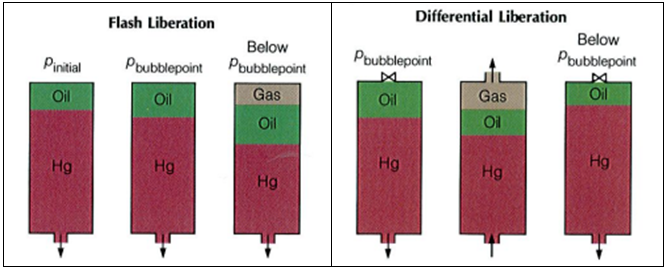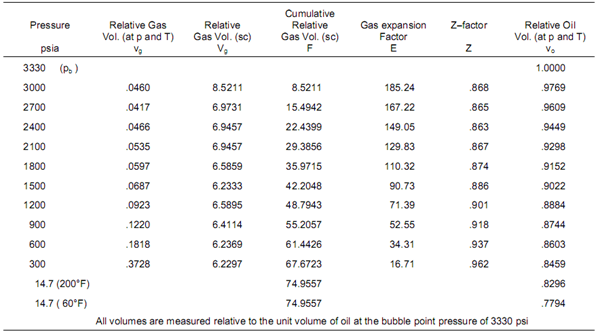# PVT Experiments: Differential Liberation

The bubble point pressure is determined by an experiment called “Constant Composition Expansion” (CCE), also called: “flash liberation”. The device used to perform this experiment is the PV cell, as shown and described in the article “Constant Composition Expansion “. The Differential Liberation (DL), discussed in this article, is experimentally performed in a similar PV cell.

The main difference between these two types of experiments is that in the Constant Composition Expansion (or flash expansion) no gas is removed from the PV cell. But instead, the gas remains in equilibrium with the oil. As a result, the overall hydrocarbon composition in the cell remains unchanged.

In the differential liberation experiment, however, pressure gradually decreases in steps and any liberated gas is removed from the oil. All depletion stages are performed at the same reservoir temperature. Therefore, there is a continual compositional change in the PV cell, the remaining hydrocarbons becoming progressively richer in the heavier components, and the average molecular weight thus increasing.The differential liberation experiment starts at the bubble point pressure determined from the CCE (since above this pressure the flash and differential experiments are identical).

### Example*:

The following example guides you on how to use and interpret the data from Differential Liberation test. The reservoir temperature is T= 200 °F and the bubble point pressure is 3330 psia.

The essential data obtained from the differential liberation experiment, performed on the same oil sample as CCE test, are listed in the following table:##### Results of isothermal differential liberation at 200 °F

After the pressure drop from 2700 to 2400 psia, column 2 of the table indicates that 0.0466 volumes of gas are taken off from the cell at the lower pressure and at 200°F. These gas volumes vg (small v) are measured relative to the unit volume of bubble point oil, as are all the relative volumes listed in the table. After each stage, the incremental volume of liberated gas is expanded to standard conditions and re−measured as Vg relative volumes (column 3). Column 4 is simply the cumulative amount of gas liberated below the bubble point expressed at standard conditions.

Dividing values in column 3 by those in column 2 (Vg/vg) gives the gas expansion factor E (column 5). Thus the 0.0466 relative volumes liberated at 2400 psia will expand to give 6.9457 relative volumes at standard conditions and the gas expansion factor is therefore 6.9457/.0466 = 149.05. Knowing E, the Z-factor of the liberated gas can be determined by explicitly solving the following equation:

Z = 35.37 × [P/(E × T)]

Z = 35.37 × [2400 × (149.05 × 660)]

Z = 0.863

These values are listed in column 6.

Finally, the relative oil volumes, vo, are measured at each stage of depletion after removal of the liberated gas, as listed in column 7.

##### * This example is from the book “Fundamentals of Reservoir Engineering”.

Primary Results from a Differential Liberation Experiment Performed on an Oil Mixture could be summarized as per below:

• Bo: Oil formation volume factor, i.e., oil volume at actual pressure, divided by volume of residual oil at standard conditions.
• Rs: Solution gas/oil ratio, i.e., the total standard volume of gas liberated at lower pressure stages than the actual one, divided by the volume of the residual oil at standard conditions.
• Oil density: Density of oil phase at cell conditions.
• Bg: Gas formation volume factor deﬁned as the gas volume at the actual pressure divided by the volume of the same gas at standard conditions.
• Z-factor gas: The compressibility factor, also known as the compression factor or the gas deviation factor, is a correction factor which describes the deviation of a real gas from ideal gas behavior.
• Gas gravity: Molecular weight of the gas liberated divided by the molecular weight of atmospheric air (= 28.964).

### You May Also Like…